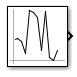# Random Number

Generate normally distributed random numbers

•Libraries:

## Description

The Random Number block generates normally distributed random numbers. To generate uniformly distributed random numbers, use the Uniform Random Number block. Both blocks use the Normal (Gaussian) random number generator (`'v4'`: legacy MATLAB® 4.0 generator of the `rng` function).

You can generate a repeatable sequence using any Random Number block with the same nonnegative seed and parameters. The seed resets to the specified value each time a simulation starts. By default, the block produces a sequence that has a mean of 0 and a variance of 1. To generate a vector of random numbers with the same mean and variance, specify the Seed parameter as a vector.

Avoid integrating a random signal, because solvers must integrate relatively smooth signals. Instead, use the Band-Limited White Noise block.

The numeric parameters of this block must have the same dimensions after scalar expansion. If you select the Interpret vector parameters as 1-D check box, and the numeric parameters are row or column vectors after scalar expansion, the block outputs a 1-D signal. If you clear the Interpret vector parameters as 1-D check box, the block outputs a signal of the same dimensionality as the parameters.

## Ports

### Output

expand all

Output signal that is the generated random numbers falling within a normal Gaussian distribution. The output is repeatable for a given seed.

Data Types: `double`

## Parameters

expand all

Specify the mean of the random numbers generated.

#### Programmatic Use

 Block Parameter: `Mean` Type: character vector Values: scalar | array Default: `'0'`

Specify the variance of the random numbers.

#### Programmatic Use

 Block Parameter: `Variance` Type: character vector Values: scalar | array Default: `'1'`

Specify the starting seed for the random number generator.

The output of number generated is repeatable for a given seed.

#### Programmatic Use

 Block Parameter: `Seed` Type: character vector Values: scalar | array Default: `'0'`

Specify the time interval between samples. The default is `0.1`, which matches the default sample time of the Band-Limited White Noise block. See Specify Sample Time for more information.

#### Programmatic Use

 Block Parameter: `SampleTime` Type: character vector Values: scalar Default: `'0.1'`

Select this check box to output a vector of length `N` if the Mean, Variance, and Seed parameters evaluate to an `N`-element row or column vector.

• When you select this check box, the block outputs a vector of length `N` if the Mean, Variance, and Seed parameters evaluate to an `N`-element row or column vector. For example, the block outputs a matrix of dimension `1-by-N` or `N-by-1`.

• When you clear this check box, the block does not output a vector of length `N` if the Mean, Variance, and Seed parameters evaluate to an `N`-element row or column vector.

#### Programmatic Use

 Block Parameter: `VectorParams1D` Type: character vector Values: `'on' | 'off'` Default: `'on'`

## Block Characteristics

 Data Types `double` Direct Feedthrough `no` Multidimensional Signals `yes` Variable-Size Signals `no` Zero-Crossing Detection `no`

## Version History

Introduced before R2006a